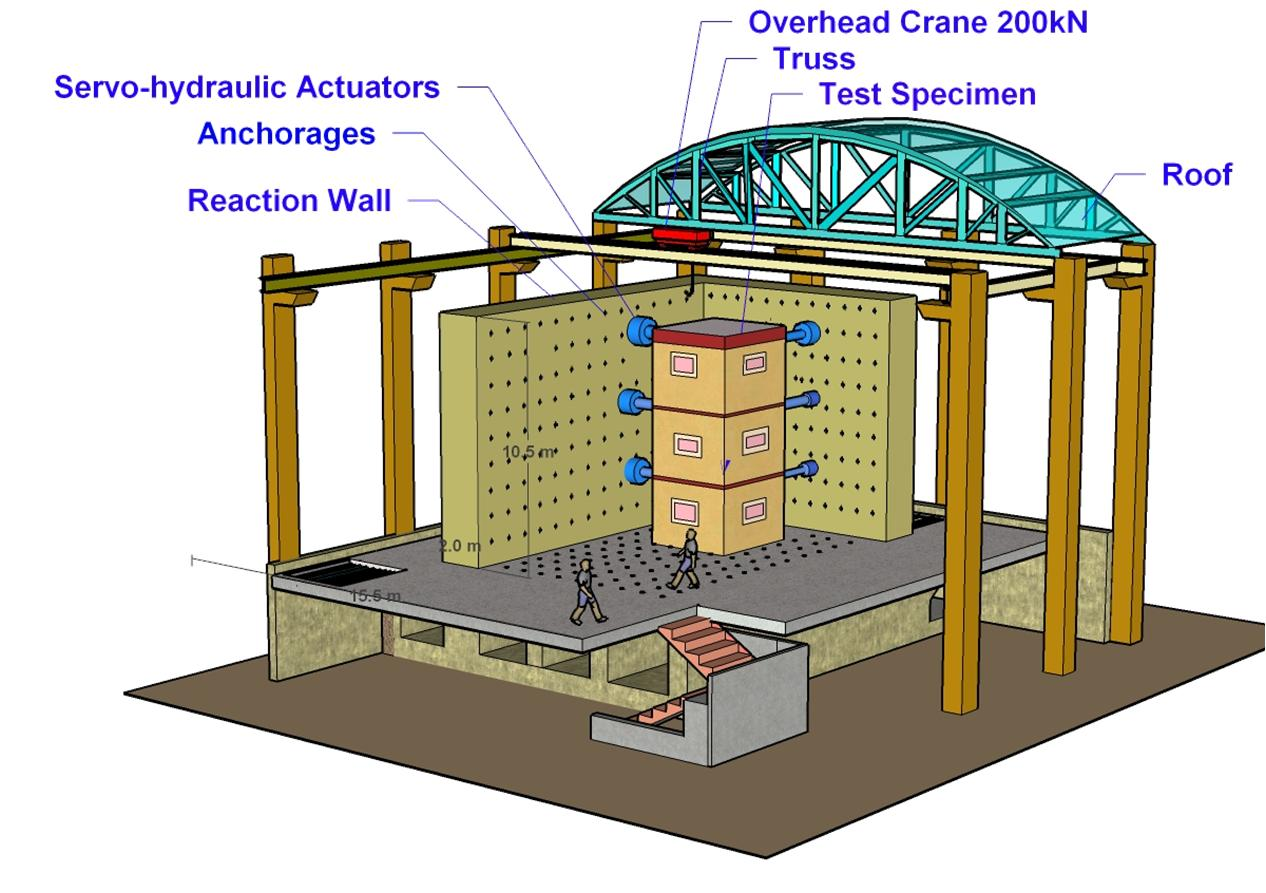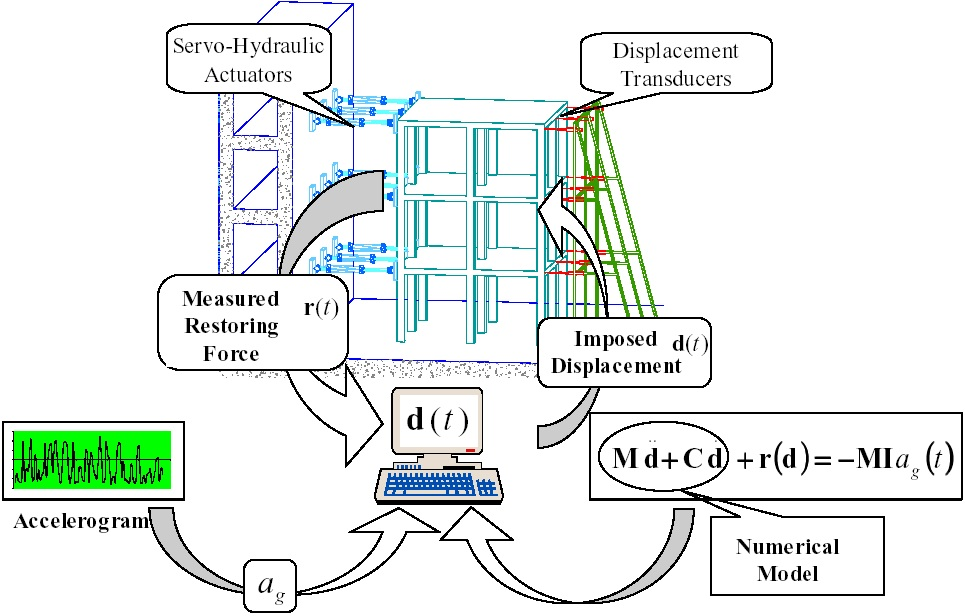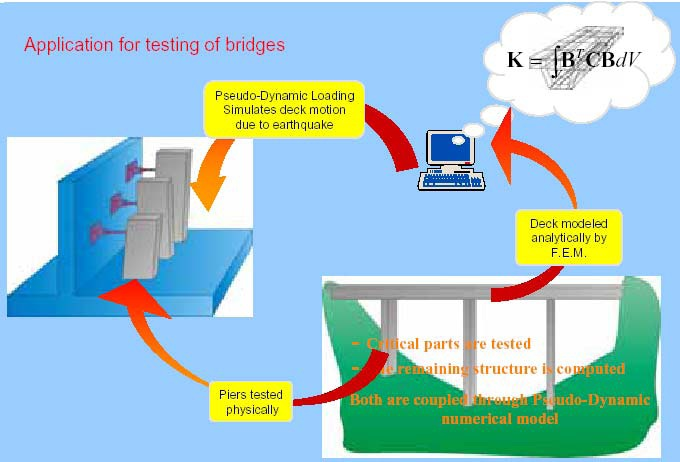Skip to main content
Feb 26, 2016

Feb 26, 2016

Feb 26, 2016

# Pseudo Dynamic (PsD) Tests - Non Linear Structural Dynamics TechniquesPDT is a substructure technique which includes applying slowly varying forces to a structural model. The motions and deformations observed in the test specimens are used to infer the inertial forces that the model would have been exposed to during the actual earthquake. The concept of pseudo-dynamic test (also called computer-actuator on-line test or hybrid test) was originated in Japan by Takanashi [Ta 75]. Since then, many researchers developed the concept and verified the suitability of this method. Quasi-static tests are simple, relatively inexpensive, and do not require very special type of apparatus.

However, the displacement history has to be defined before the test, which is the main limitation of this testing technique. Similarly, the applied cyclic displacement history may not cover the range of displacements, which the structure would undergo under dynamic action.

The basic concept of pseudo-dynamic test is that the dynamic response is computed using the experimental result in each time step. During the analysis process, the computer calculates the structural response (displacement) in a time step.

Inertial and damping forces, required during the analysis process, for the solution of the equations of motion are modeled analytically. The computer, after calculating structural displacement at a specific time step, electronically provides this result to the actuator system. In the experimental process, actuator control system imposes the calculated displacement and then measures and returns the restoring force, R(t), to the computer. With the measured data, the computer can calculate the response in the next time step. With this feedback procedure, the nonlinear inelastic dynamic response can be obtained without shaking table test devices. The flow of this feedback is shown in Figure below.The method has difficulty in idealizing infinite degrees of freedom as a few degrees of freedom. However, it enables a dynamic test with a static test device, which attracts many researchers. The process automatically accounts for the hysteretic damping, due to inelastic deformation and damage to the structural materials, which is usually the major source of energy dissipation. Inertia forces are not experimentally produced and are modeled numerically. This eliminates conducting the test on a real time-scale, and allows very large models of structures to be tested with only a relatively modest hydraulic power requirement.## Advantages and Disadvantages of PsD Testing:

Structures larger than a laboratory itself such as bridges, towers etc., can also be tested by means of the PDT method exploiting the substructuring technique. This procedure tests only the most critical part of the structure experimentally and the lets the rest of the structure be modelled analytically.

Another major advantage of this technique over the quasi-static testing is the use of a special procedure of pseudo dynamic test known "sub-structuring". Taking advantage of this technique, researchers can test only a part of the structure. The rest of the structure can be analytically modeled on a host computer. e.g., one can model a bridge deck analytically on a host computer and can carry out the test on the bridge piers by pseudo dynamic testing facilities (image above). This saves substantial amount of cost and time related to testing work.

A major drawback of PsD testing technique is that a lot of time is consumed to conduct the experiment. This is mainly due to the time required by computer hardware to solve the equations of motion for determining the displacements and by the control system to execute the calculated displacements. Simulation of an earthquake, lasting 20 seconds, takes hours of time with this technique. As a result, the response determined by this test of the structures that are sensitive to loading rate (such as masonry) becomes questionable.

Another drawback is, that due to lumped mass idealization, the testing method is not adequate for structures with distributed mass, e.g., hydraulic structures. To test such types of structures, a refined spatial discretization would be required resulting in a large number of actuators. This reduces the effectiveness of testing for such structures. The applicability of this method also relies on the appropriate assignment of damping properties. It has been observed that the use of constant damping matrix based on the elastic properties of the system resulted in unpredictable results.

## Real time Pseudo Dynamic Testing:

RTPD test technique is same as the PSD test except that it is conducted in the real time. It introduces the problems in control, such as delay caused by numerical simulation and actuator.

Let us know in the comments what you think about the concepts in this article!